# What is a Vector? – Explanation & Examples

Vectors efficiently convey information about a mathematical or physical element. In particular:

Vectors are mathematical quantities used to represent objects that have both magnitude and direction.

Have you ever wondered what makes speed different from velocity or mass different from weight? Hint: The answer is related to vectors! We will explore these questions and more as we discuss the following vector topics in this article:

• Vector Definition
• Introduction to Vectors

## Vector Definition

In physics and mathematics, a vector is defined as:

“An object or the physical quantity which can be represented by both magnitude and direction.”

Using the above definition, we can see that the representation of vectors requires the presence of two components, namely:

• Magnitude (or size)
• Direction

## Introduction to Vectors

Historically, vectors were used in geometry, physics, and mechanics.  However, as time has passed, vectors have become widely used in many fields, including linear algebra, engineering, computer science, structural analysis, and navigation.

Since vectors express two notions, namely magnitude, and direction, they can construct a wide variety of mathematical models for various problems and scenarios.

In this section, we will learn about the following important vector concepts:

• Geometric and Mathematical Representations of Vectors
• Scalars vs. Vectors
• Different Types of Vectors

### Geometric and Mathematical Representation of Vectors

Vectors can be geometrically represented by straight arrows of a specific length pointing in a specific direction with specific starting and ending points. The vector’s length represents its magnitude, whereas the direction indicates its direction concerning a set of coordinates. The image below is an example of a geometric representation of a vector.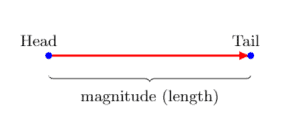Consider the following figure where A is a vector. |A| represents its length (or magnitude), and the arrowhead pointing from point a to point b represents its direction. Point a is called the initial, or starting, point, and point b is called the terminal or ending point of the vector A. Although this example shows a vector in two dimensions, it can also have three, four, or higher dimensions.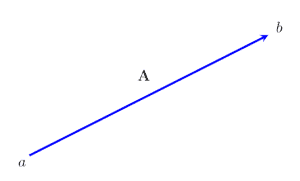The magnitude of the vector is basically the same as the length of the line segment ab. The direction of the vector is basically the same as the direction of the arrow.

Algebraically, a vector can be expressed as an ordered pair. This representation is called a column vector. In the image below, the vector OA is represented as a column vector.

OA = (2,3)

This means that the vector is displaced from the origin by two points along the horizontal (x-axis) and four points along the vertical axis (y-axis).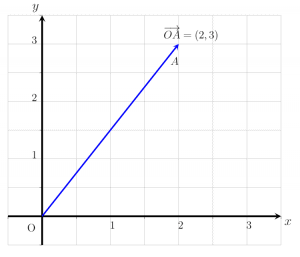Vectors are often represented by boldface letters like a or A. If boldface is not possible, such as when writing notes by hand, a vector is represented by a letter with an arrowhead above it.

### Vectors vs. Scalars

Physical and mathematical quantities are classified as either vectors or scalars. Though they are related, vectors and scalars are used in different situations.

Scalar Quantity

A scalar quantity has magnitude but no direction.

Scalars are represented by simple letters like a or A, and they typically consist of real numbers. Some common examples of scalars are time, speed, energy, mass, volume, area, and height.

Vector Quantity

A vector quantity has both magnitude and direction.

Unlike scalar quantities, which have only one component, vector quantities consist of two components. Some common examples of vectors include velocity, displacement, and acceleration.

To better understand the difference between scalar and vector quantities, let’s consider a few examples:

Identify whether the given quantity is a vector or a scalar.

V = 10m, East

To classify this quantity, we need to consider the definitions of vectors and scalars and figure out how many components it has. We first decompose the given quantity into its parts. The given quantity has a magnitude component of |V| = 10m. It is also pointing towards the East. Therefore, we can conclude that the given quantity is a vector because it has two component parts.

A = 5 cm

In this example, only the magnitude component is present. Since there is no mention of a direction, this quantity is a scalar.

The magnitude of scalar A is given as 5 cm.

### Different Types of Vectors

Different types of vectors used in mathematics include:

• Zero Vector
• Unit Vectors
• Equal Vectors
• Displacement Vectors
• Negative of a Vector
• Position Vectors
• Co-initial Vectors
• Collinear Vectors
• Coplanar Vectors

Each of these types of vectors is very important and has various applications. Their descriptions can be found below.

#### Zero Vector

A vector is called a zero vector if its magnitude is zero. A zero vector starts and ends at the same point, which means it has the coordinates (0,0). It also has no specified direction. For example:  A = (0,0) and  A = 0 are different ways of writing zero vectors.

#### Unit Vector

A unit vector is a vector whose length or magnitude is 1. Finding a unit vector with the same direction as another vector can be a useful tool, and we call this a normalized vector. Such a vector is found by dividing the given vector by its magnitude:

Y hat = Y/ |Y|

Note: Remember that unit vectors are only equal to each other if they point in the same direction.

#### Equal Vector

Two or more vectors are said to be equal if they have the same magnitude and point in the same direction. The two vectors, A and B, in the image shown below are equal since their magnitude and direction are the same.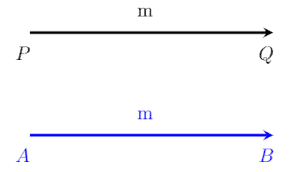#### Displacement Vector

If point X is displaced (moved) from one position to another position, Y, then the displacement between two points can be represented in the form of a displacement vector. In this case, the displacement vector would be written as XY.

#### Negative of a Vector

Two vectors with the same magnitude but opposite direction are called the negatives of each other. Let a and b are two vectors with the same magnitude. If the direction of b is opposite to that of a, then a and b are the negatives of each other. The relationship between these two vectors is:

a = -b

#### Position Vector

The position vector is used to indicate an object’s position in three-dimensional Cartesian coordinates concerning a specified reference point.

#### Co-initial Vectors

Two or more vectors having the same initial or starting point are called co-initial vectors. In the image given below vectors, AC and AB are co-initial vectors.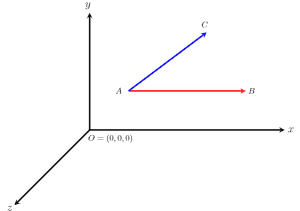#### Collinear Vectors

Vectors that are parallel to each other or which lie on the same line are called collinear vectors.

#### Coplanar Vectors

Two or more three-dimensional vectors that lie in the same plane are called coplanar vectors.

### Examples

In this section, we will discuss some vector example problems and their step-by-step solutions.

Example 1

Express the given vector AD as shown in the image below as a column vector.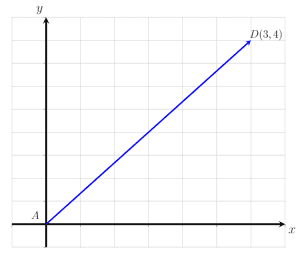Solution

By definition, the column vector is expressed as an ordered pair. It is clear from the figure that AD starts at point A and ends at point D. It is displaced 3 units towards the right along the x-axis and 4 units upward along the y-axis.

Thus, the given vector AD written as a column vector is:

Example 2

Express the given vector UV as shown in the image below as a column vector.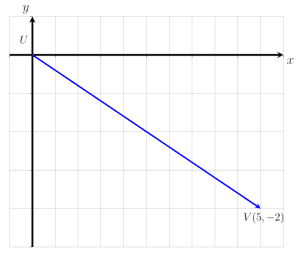Solution

By definition, the column vector is expressed as an ordered pair. It is clear from the figure that UV starts at point U and ends at point V. It is displaced 3 units to the right along the x-axis and 2 units downwards along the y-axis.

Thus, the given vector UV written as a column vector is:

UV = (5, -2)

Note that the negative sign indicates that the vector’s movement is downwards along the y-axis.

Example 3

Identify the given quantity as scalar or vector.

S = 40 minutes

Solution

The given quantity is a scalar because it only has magnitude and no direction. Its magnitude is |S| = 40.

Example 4

Identify the given quantity as scalar or vector.

OW = (2,-3)

Solution

The given quantity is a vector. It is expressed as a column vector, OW, where O is the starting point, and W is the terminal point. This shows that the translation from O to W is 2 points to the right along the horizontal axis and 3 points downwards along the y-axis.

Example 5

Identify the given quantity as scalar or vector.

V = 0

Solution

The given quantity is a vector. The magnitude of the vector V is given as |V| = 0, so this is actually a zero vector. The direction of this vector is therefore unspecified since the zero vector does not have a direction.

Example 6

Identify the given quantity as scalar or vector.

F = 20N, down

Solution

The given quantity is a vector. The magnitude of the vector, F, is |F| = 20, and the direction is given as downward.

### Practice Questions

Identify the following quantities as vectors or scalars and determine both their magnitudes and directions.

1. X = 2m, North
2. X = 250 Kg
3. F = 20N, Upward
4. V = 30 m/s, West
5. T = 20 sec
6. Y = (3,2)
7. A = 10 m/s^2 , vertically up.
8. S = 20cm at 60 degrees
9. W = (2,5)
10. V = 20 mph, North East
11. Express the given vector PQ as shown in the image below as a column vector.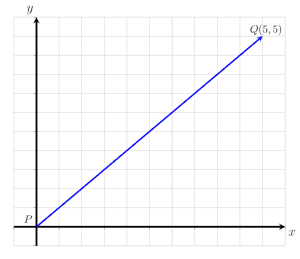12. Express the given vector MN as shown in the image below as a column vector.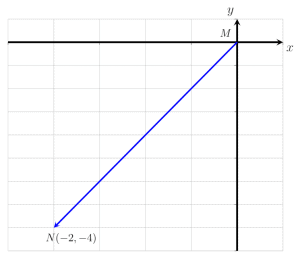1. Vector: Magnitude is|X| = 2m, and direction is given as north.
2. Scalar: |X| = 250Kg, and only the magnitude is given.
3. Vector: Magnitude is |F| = 20N, and direction is given as upward.
4. Vector: Magnitude is given as |V| = 30 m/s, and direction is given as West.
5. Scalar: |T| = 20, and only magnitude is given.
6. Vector: It is a column vector where 3 represents 3 points to the right along the x-axis, and 2 represents 2 points upward along the y-axis. Magnitude is given as |Y| = sqrt(3^2 + 2^2)
7. Vector: Magnitude is given as |A|= 10m/s^2, and direction is upwards.
8. Vector: Magnitude is |S| = 20cm, and direction is at an angle of 60 degrees.
9. Vector: This column vector moved 2 points to the right along the horizontal axis and 5 points upwards along the vertical axis. Magnitude is given as |W| = sqrt(2^2 + 5^2)
10. Vector: Magnitude is |V|= 20 mph, and direction is given as North East.
11. The vector, PQ, can be expressed as the ordered pair:

PQ = (5,5).

This means the vector PQ starts at point P and ends at point Q. It is translated 5 points to the right along the horizontal axis and 5 points upwards.

1. The vector, MN, can be expressed as the ordered pair:

MN = (-2, -4).

This means the vector MN starts at point M and ends at point N. It is translated 2 points to the left along the horizontal axis and 4 points downwards along the y-axis.